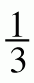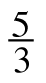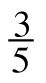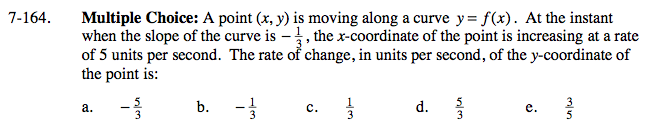### Home > CALC > Chapter 7 > Lesson 7.3.5 > Problem7-164

7-164.
1. Multiple Choice: A point (x, y) is moving along a curve y = f(x). At the instant when the slope of the curve is −, the x-coordinate of the point is increasing at a rate of 5 units per second. The rate of change, in units per second, of the y-coordinate of the point is: Homework Help ✎

1.2.3.4.5.$\text{Slope}=\frac{\text{rise}}{\text{run}},\text { where run is the change in the }x\text{-coordinate and rise is the change in the }y\text{-coordinate.}$

Solve for rise.

$\text{slope}=-\frac{1}{3}=\frac{\Delta y}{\Delta x}$

$-\Delta x=3\Delta y$

Implicitly differentiate with respect to t.